# Watson Studio

View Only

### k-fold Cross-validation in IBM SPSS Modeler

#### By Archive User posted Wed March 02, 2016 06:21 PM

There are many different ways that one can evaluate the accuracy and stability of a predictive model and IBM SPSS Modeler natively includes support for several of these.

The most commonly used method that is supported in Modeler is the "Holdout Method". In this method, the dataset is randomly partitioned into 2 (or sometimes 3) independent sets:

1. A training set that is used for training or estimating the model

2. A test set that is used for testing, refining, and selecting the model

3. An optional validation set that is used for validating and evaluating the selected model

The 'Partition' node from the 'Field Ops' palette provides the primary support for this method.

A number of the modeling techniques also support "bagging", short for "Bootstrap Aggregation", which utilizes the "Bootstrap Method" to estimate a number of models on samples of the dataset.

However, many data scientists are using the "Cross-validation Method" which is not supported in SPSS Modeler without a little extra work. The objective of this article is to describe a way in which one can implement the "Cross-validation Method" in SPSS Modeler.

# What is the "Cross-validation Method"?

The most common method is the k-fold cross-validation. Here the initial dataset is first partitioned randomly into a number (k) of subsets with an approximately equal number of records in each subset. Each subset is then used as the test partition, while the remaining subsets are combined to perform the role of the training partition. This produces k models that are all trained on different subsets of the initial dataset and where each of the subsets have been used as the test partition exactly once.

Model 1 Model 2 Model 3 Model ... Model k
Fold 1 Testing Training Training ... Training
Fold 2 Training Testing Training ... Training
Fold 3 Training Training Testing ... Training
Fold ... ... ... ... ... ...
Fold k Training Training Training ... Testing

The estimated accuracy of the models can then be computed as the average accuracy across the k models.

There are a couple of special variations of the k-fold cross-validation that are worth mentioning:

• Leave-one-out cross-validation is the special case where k (the number of folds) is equal to the number of records in the initial dataset. While this can be very useful in some cases, it is probably best saved for datasets with a relatively low number of records.

• Stratified k-fold cross-validation is different only in the way that the subsets are created from the initial dataset. Rather than being entirely random, the subsets are stratified so that the distribution of one or more features (usually the target) is the same in all of the subsets.

The choice of the number of fold to use in the k-fold cross validation depends on a number of factors, but mostly on the number of records in the initial dataset:

• When working with very sparse datasets, a high k - even to the point of the leave-one-out (k=n) - can be beneficial

• When the initial dataset has a high number of records, a lower number of folds can be quite accurate

• The most common choice for k is 10. This generally seems to provide the best balance between processing time and accuracy.

There is plenty of available literature that describes these methods in much more detail.

# k-fold Cross Validation in SPSS Modeler

For the sake of simplicity, I will use only three folds (k=3) in these examples, but the same principles apply to any number of folds and it should be fairly easy to expand the example to include additional folds.

Sample IBM SPSS Modeler Stream:  k_fold_cross_validation.str

I am using one of the sample data sets that come installed with IBM SPSS Modeler: "tree_credit.sav". This is the data set that is used in the Introduction to Modeling tutorial, where the data is also described in a little more detail:

The bank maintains a database of historical information on customers who have taken out loans with the bank, including whether or not they repaid the loans (`Credit rating = Good`) or defaulted (`Credit rating = Bad`). Using this existing data, the bank wants to build a model that will enable them to predict how likely future loan applicants are to default on the loan.

## Assign Each Record to a Fold

The first step is to assign each record in the data set to exactly one of the three folds. This is done at random using a 'Derive' node to create a new field named `Fold` with the formula: `random( 3 )`, where 3 is the number of folds that I will be using in the cross validation. This assigns an integer value between 1 and 3 to each record.

[caption id="attachment_4149" align="aligncenter" width="350"]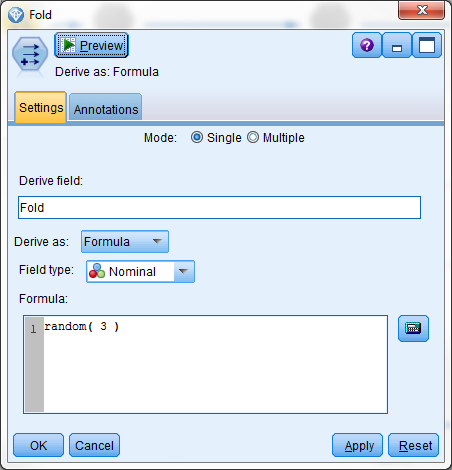Figure 1 - Assigning a fold to each each record at random[/caption]

One very important thing to keep in mind here is that this assignment is random and that it is done each time the data passes through the node and this means that the assignment will be different every time a node is executed. The 'Partition' node that is normally used for the Hold-out Method has an option to enforce "Repeatable partition assignment", but there is no such option for the `random()` function.

There are different ways to resolve the issue of repeatable assignment:

• Using a Cache.  The simplest option (and the one used in this example) is to enable a Cache on the node that assigns the Fold. This will ensure that the assignment (along with the rest of the data set) is stored in a temporary file or database table that will be used for the remainder of the session once it has been filled. This adds a small icon to the top-right corner of the node, which turns green when the cache is filled.

The assignment, however, will not persist across sessions and the records will be reassigned every time the stream is closed and reopened or if the cache is flushed for some other reason. For data sets with a larger number of fields, this may also result in an unnecessarily large temporary file as it essentially creates a copy of the complete data set.

You can find more details in the Caching Options for Nodes article.

• Using an Export.  The other option would be to create the assignment once and then export it to a file or a database table along with one or more fields that will uniquely identify each record. The exported assignment can then be merged with the original source data for an assignment that will persist across sessions.

When working with a data set where new records may be added over time, there must be a mechanism that allows the assignment of a fold to these new records, while still maintaining the assignment of the existing records.

For the examples in this article, I will be utilizing the Cache method and since I am using a file based data source, the cache will also be file based as indicated by the small, green file icon that is added to the node when the cache is filled.

[caption id="attachment_4159" align="aligncenter" width="300"]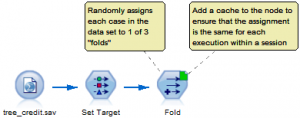Figure 2 - Using a file-based cache[/caption]

## Train a Model for Each Fold

For each fold, the process of setting up and training the model is then the same:

 Model ID. Create a field that indicates the `Model ID`, so that it is possible to identify the model that the record was passed through later. I have used the numbers 1, 2, and 3 to identify the models in the example. Derive Partition. Derive a field that will be used as to partition the data set into training and testing partitions based on the assigned fold for each record. I have utilized that my Model IDs and the fold assignments are both the numbers 1, 2, or 3 to set the records where the `Fold` is equal to the `Model ID` as the testing partition and the remaining records as the training partition. [caption id="attachment_4160" align="aligncenter" width="350"]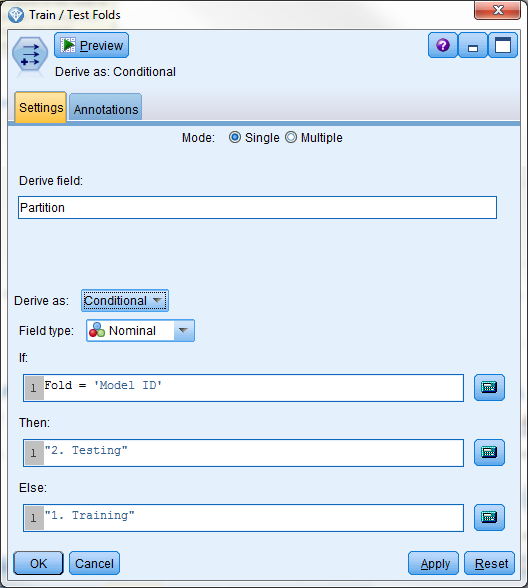Figure 3 - Deriving the Training and Test folds[/caption] This field must have the type "Nominal" or "Ordinal" to be used in the role of "Partition". When the partitioning is created in this way, Modeler will use the first value as the training partition and the second value as the testing partition. This is why it is common to add a number as the first part of the value to ensure that the correct partition is used. You can find more details in the Setting the Field Role article from the SPSS Modeler Help. Set Role. Set the role of the field to "Partition" using a 'Type' node. Train Model. Use an appropriate model trainer node to train a model. Make sure to check the "Use partitioned data" option on the "Model" tab if this option is available, but most of the model trainer nodes will either always pick up the partition role and use or have the option checked by default. It is important that all of the settings in the model trainer nodes are the same for each fold. The objective is to determine the stability of the model and not to determine if different settings will result in a more accurate or suitable model. This will create a model applier node that the source data can be passed through for scoring.

This leaves us with three separate models in the example.

[caption id="attachment_4167" align="aligncenter" width="800"]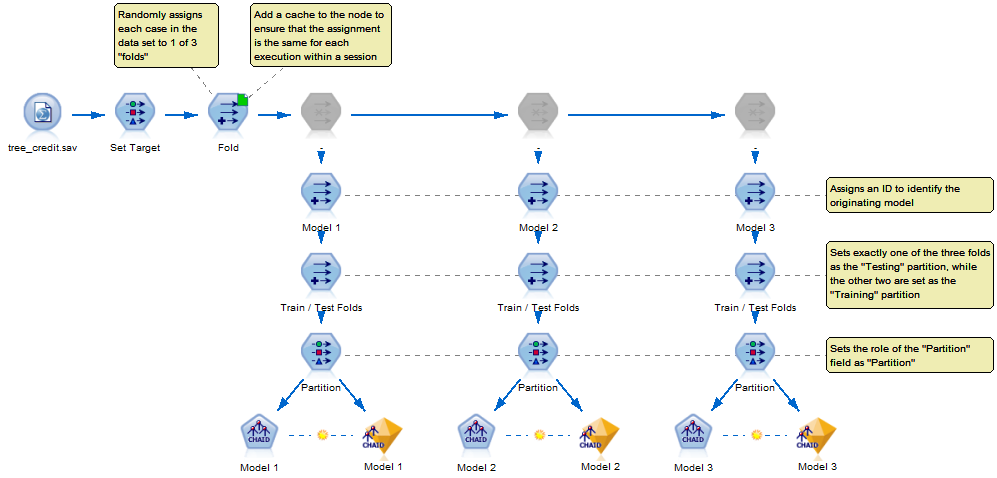Figure 4 - SPSS Modeler stream with the 3 component models[/caption]

The three gray nodes along the top of the stream are 'Filter' nodes that have been disabled. They have no effect on the data that passes through them and are only used as a way to organize the stream and to ensure that the connections do not cross other connections or through any nodes.

Please notice that I have deliberately selected the modeling options so that the resulting models are sub optimal. This is done to ensure that we will be able to tell the models apart when evaluating the results later.

## Combine the Model Results for Evaluation

The results or estimates produced by the different models can easily be viewed individually, but in order to make a direct comparison between the models, the results need to be combined and viewed together.

[caption id="attachment_4172" align="aligncenter" width="800"]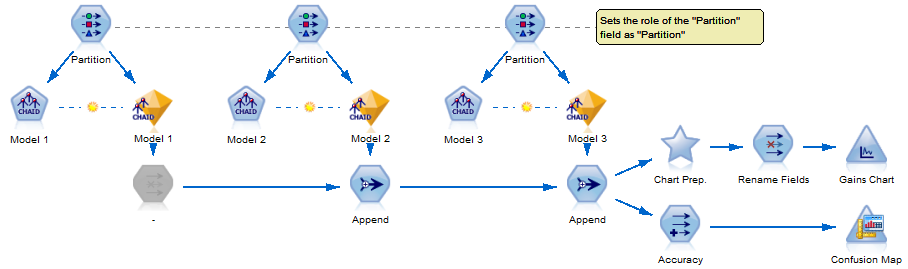Figure 5 - Combining the component models for evaluation[/caption]

The entire data set is passed through each of the model appliers and combined using one or more 'Append' nodes to form a data set where each record appears exactly k times. Technically, any number of data sets can go into a single "Append" node, but I prefer using multiple simply because I think it makes for nicer looking flow when the arrows do not cross each other or any nodes.

The nodes that are regularly used for evaluating the models such as the 'Analysis' node and the 'Evaluation' node are not immediately equipped to handle and compare a number of component models; they are set up to compare the results from multiple models using different algorithms and settings, but all using the same records for the training and testing partitions. Here we have multiple models all with the same algorithm and settings, but using different records for the training and testing partitions.

There are many ways to compare the results of the component models, but one popular method for models with a binary target is the Gains Chart. This is normally a part of the 'Evaluation' node, but because for this purpose we will have the recreate the chart using more basic functionality. The 'SuperNode' named "Chart Prep." uses a number of parameters that can be set by editing the node to indicate the name of the fields that have the different required roles. This also requires that the propensity score is made available since this is used in the chart.

[caption id="attachment_4174" align="aligncenter" width="450"]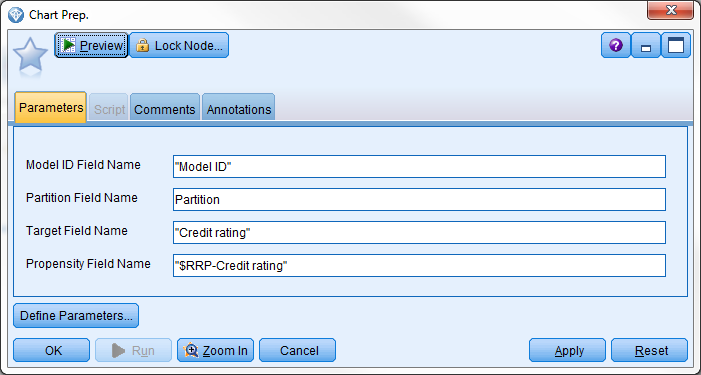Figure 6 - The parameters from the "Chart Prep." SuperNode[/caption]

The chart that is produced is similar to that from the normal 'Evaluation' node and can be read in the same way. In the Gains Chart shown below it can be seen that the gains from each of the component models are represented by a colored line.

[caption id="attachment_4176" align="aligncenter" width="800"]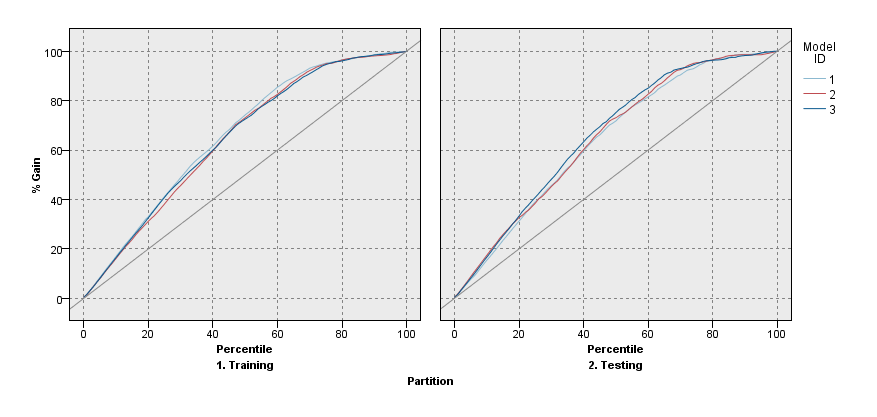Figure 7 - Gains Chart showing the three component models[/caption]

For this example, the lines pretty much fall in top of each other indicating that the model is not sensitive to how the records are partitioned into the training and testing partitions, which is what you would want to see.

# Stratified Cross Validation

As mentioned at the beginning, the only difference between the k-fold cross validation and the stratified cross validation is the method used for assigning records to each of the folds.

Where the method used in the k fold cross validation is random, the method used by the stratified cross validation is stratified - not surprising since it is right there in the name.

In the example, there is not a real need for employing stratification as the target is fairly balanced to start with and the resulting folds seem to retain that balance without stratification, but for targets that have imbalanced distributions, stratification can be vital.

## Simple Stratification

With just two categories for the target field, the stratification can be done by splitting the data set by the value of the target field and assign the fold at random within each subset of the data. This should ensure that roughly the same number of records with Bad credit is assigned to each of the three folds and similarly for the customer with Good credit.

Sample IBM SPSS Modeler Stream:  stratified_folds_simple.str

[caption id="attachment_4179" align="aligncenter" width="542"]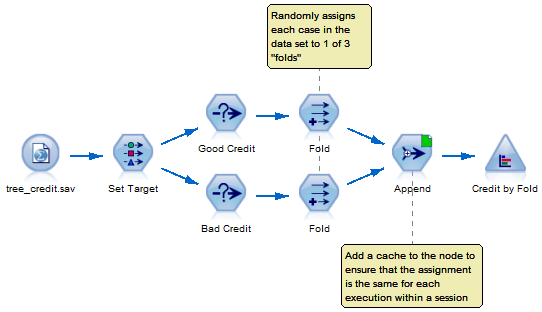Figure 8 - Simple stratification[/caption]

The example only has two categories for the target field, but it is easy to expand this method for use with targets that have more than 2 categories by adding a copy of the 'Select' node and the node that assigns each record to a fold.

Since the assignment is random, the number of records in each fold will not be exactly the same and if it is important that the numbers are exact or if the data set has a low number of records then a different method might be required.

## Exact Stratification

The approach above can be expanded slightly to ensure that the number of records in each fold is exactly the same for each category of the target field.

Sample IBM SPSS Modeler Stream:  stratified_folds_exact.str

[caption id="attachment_4181" align="aligncenter" width="800"]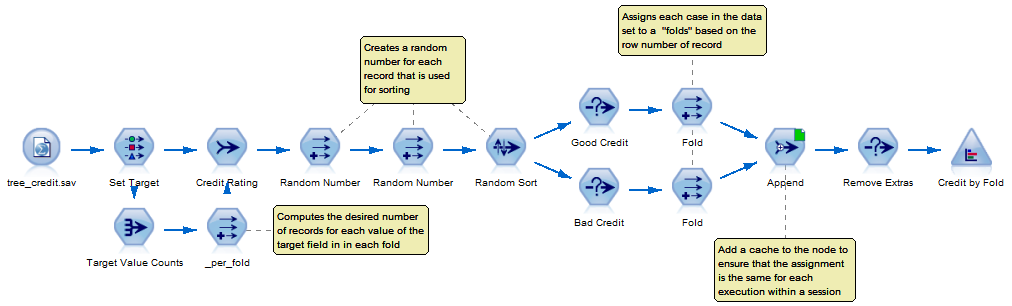Figure 9 - Stratification with exact numbers in each fold[/caption]

First the desired number of records for each of the values of the target field (`Credit rating`) is computed by taking the total number of records in each category (in the 'Aggregate' node named "Target Value Counts") and then dividing that number by the number of folds (k) and rounding it down to the nearest integer (in the 'Derive' node named "_per_fold").

The records are then shuffled to ensure that their order in the data set is random. This is done by creating two random numbers that are then used for sorting the data set. The first random number is used for the primary sorting and the second is used as a kind of a tiebreaker in case two or more records have the same number assigned by the first one.

The data set is then split by the value of the target field as before, but the assignment of the fold is not done at random. It is done based on the row number of the record in the split data set, which is now random. This can be done in several different ways. The one used in the example can be used regardless of the number of folds and the formula used in the same in both of the 'Derive' nodes named "Fold".

[caption id="attachment_4182" align="aligncenter" width="450"]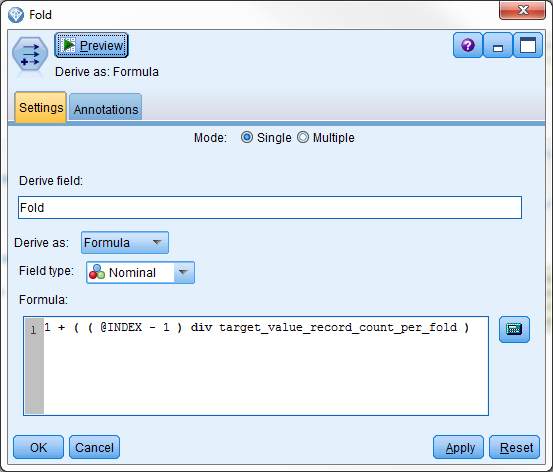Figure 10 - Computing the fold[/caption]

The formula refactors the row index to start at 0 rather than 1 and then performs an integer division between the refactored row index and the desired number of records for the target value in each fold. It finally adds 1 to the result since we want the ID of the folds to start at 1 and not 0.

The "Remove Extras" node discards any records that are assigned to a fold with an ID that is greater than 3. This happens if the number of records in target category is not an exact multiple of the number of folds (k).

## Complex Stratification

There may be some cases where it is useful to ensure that the folds have the distribution of more fields than just the target field and the methods described above do not allow for that.

IBM SPSS Modeler includes a 'Sample' node and this allows for stratified sampling of records and this can be exploited as we need to a stratified sample of the records in each fold. The 'Sample' node also has the option to enable "Repeatable partition assignment", so that the sampling will give the same results for as long as the source data does not change - both within a session and across sessions.

Sample IBM SPSS Modeler Stream:  stratified_folds_complex.str

[caption id="attachment_4183" align="aligncenter" width="800"]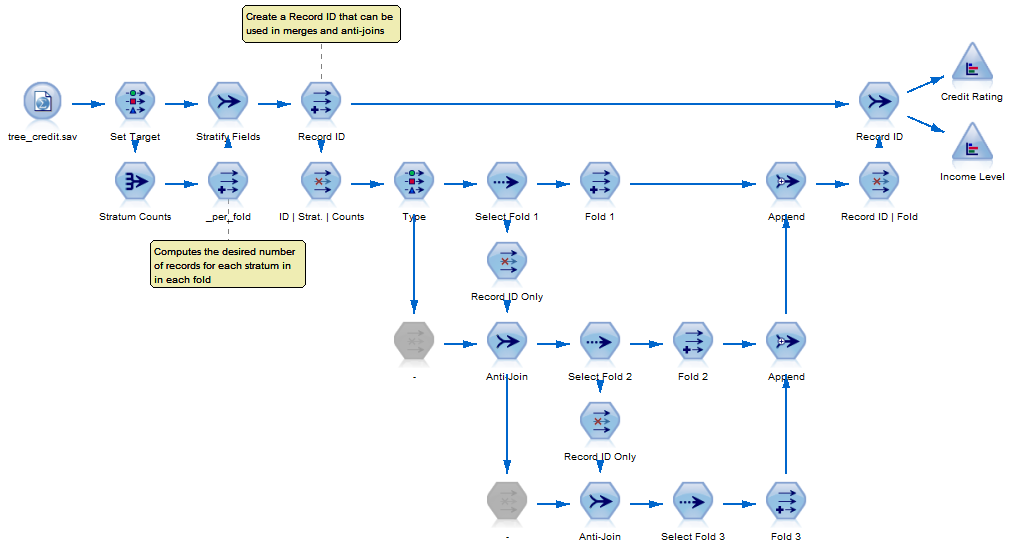Figure 11 - Using complex sampling to create the stratified folds[/caption]

The desired number of records in each stratum is computed in the same way as above, but this is no longer limited to the just include the target field, but can include any relevant categorical field from the data set.

The sampling has to be done one fold at a time while making sure that the sampling is done without replacement to ensure that a record can only appear in exactly one fold. With just 3 folds the process is fairly simple as shown above, but a section of this stream must be repeated for each fold and the settings of all of the 'Sample' nodes must be identical.

The fields that are used in the stratification must be selected in several places for this to work:

• As the "Key fields" in the 'Aggregate' node named "Stratum Counts".

• As the "Keys for merge" in the 'Merge' node named "Stratify Fields" and is set up as an anti-join.

• They must be included as output fields in the 'Filter' node named "ID | Strat. | Counts".

• The fields must be instantiated in the 'Type' node. This is a requirement for the 'Sample' node to work correctly.

• As the "Stratify by" fields in each of the 'Sample' nodes under the "Cluster and Stratify" option when the "Complex" method is selected.

#Usecases
#SPSSModeler
#spss
14 views

Wed September 26, 2018 03:51 AM

Tue July 24, 2018 04:13 PM

Hi, I´m new using modeller. I need to do a Leave-One-Out Cross Validattion (LOOCV). How is possible to do it? Because in the example is done a 3-fold cross validation and I don´t get how to do it for LOOCV.
Thanks!

Thu October 13, 2016 08:16 PM

I was looking for this exact thing. Very useful.

Fri March 11, 2016 10:37 AM

Thank you for posting this very good article

Wed March 09, 2016 10:29 AM